# Generate MATLAB Code for Sensitivity Analysis Statistics to Identify Key Parameters (GUI)

This example shows how to automatically generate a MATLAB function to solve a Sensitivity Analysis statistics problem. You use the Sensitivity Analyzer to define a sensitivity statistics problem for a model of the body's vestibulo-ocular reflex, and generate MATLAB code to solve this statistics problem.

### Vestibulo-Ocular Reflex Sensitivity Statistics Problem

The Identify Key Parameters for Estimation (GUI) example shows how to use the Sensitivity Analyzer to compute sensitivity statistics for different parameter values in a model of the body's vestibulo-ocular reflex. In this example, we load a preconfigured Sensitivity Analyzer session based on that example.

Open the Sensitivity Analyzer for the sdoVOR model:

`ssatool('sdoVOR')`

In the Sensitivity Analyzer, click Open Session and `Open from model workspace`. Open session `sdoVOR_sasessionForSensitivityStatistics`.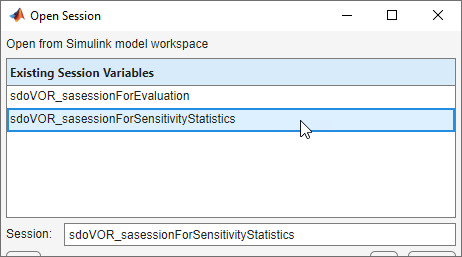This opens a preconfigured session in the Sensitivity Analyzer.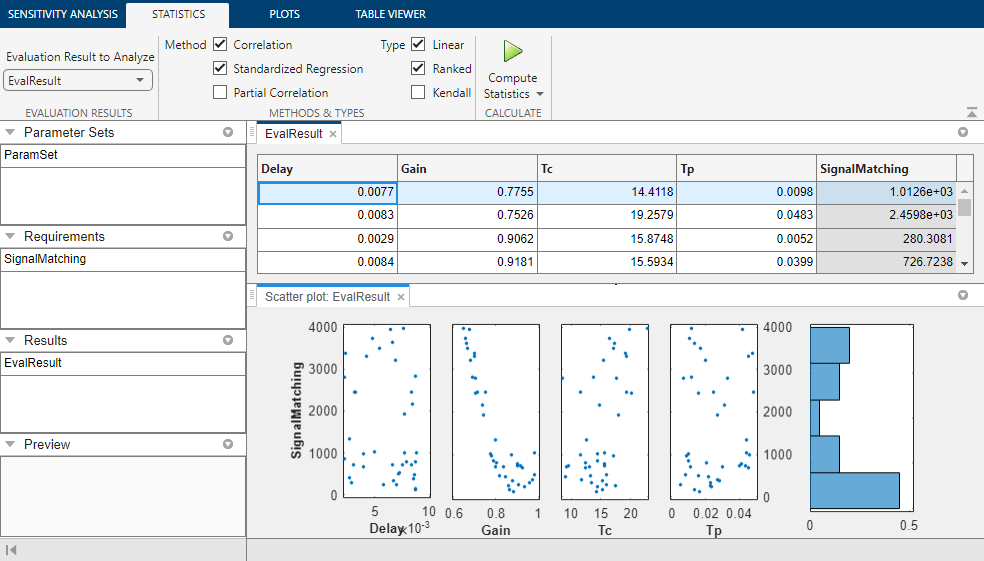### Generate MATLAB Code

From the Compute Statistics list, select `Generate MATLAB Function`.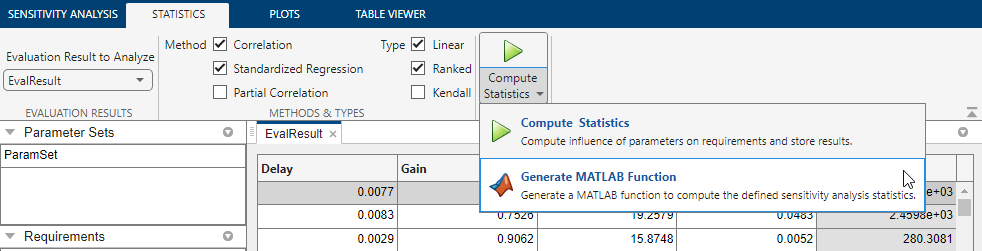The generated code is added to the MATLAB editor as an unsaved MATLAB function.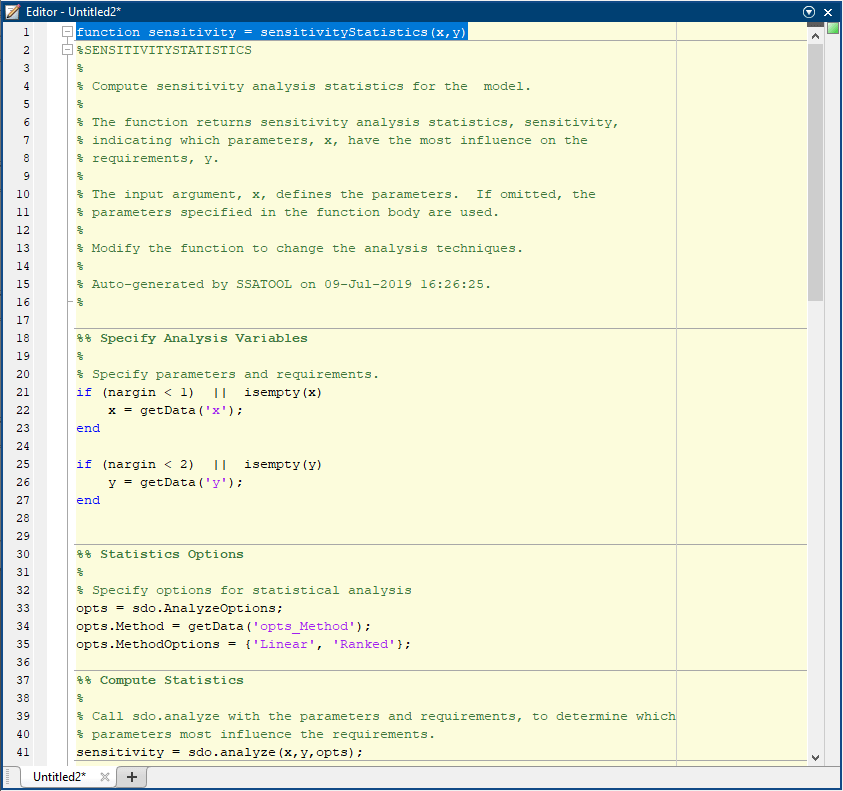Examine the generated code. Significant portions are:

• Statistics Analysis Variables - Specify the inputs and outputs, to determine which inputs have the most influence on outputs.

• Statistics Options - Specify the types of analyses to be computed.

• Compute Statistics - Solve the sensitivity statistics problem using the sdo.analyze command.

Select Save from the MATLAB editor to save the generated function.

### Run Generated Code

Run the generated function.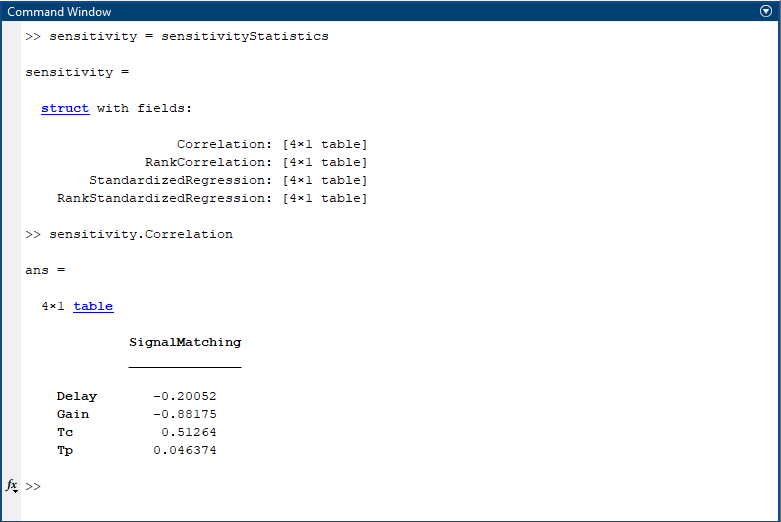The computation shows the result of analyzing which inputs have the most influence on the output. For example, the `Correlation` field shows that the `Gain` parameter has the largest magnitude correlation with the output, and in a negative direction, meaning that when Gain increases, the output decreases.

### Modify the Generated Code

You can:

• Modify the specified input and output variables.

• Modify the options to change the types of analyses computed.

## Related Topics